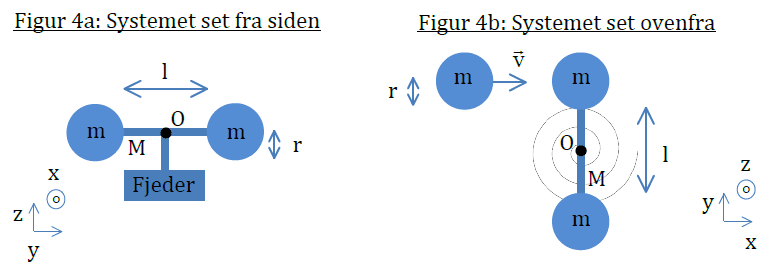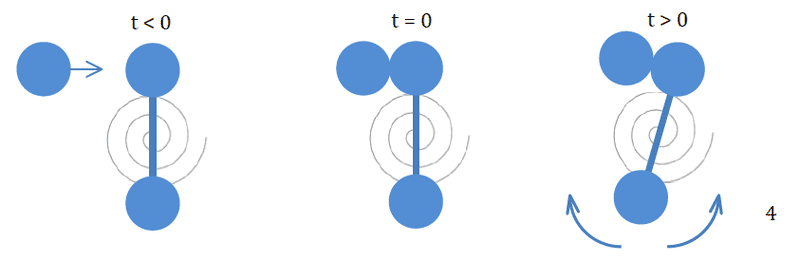# Torque that changes angular moment -- Help please

I need to find the maximum contortion of a torsion spring, for a given system. Most of the assignment I have done correct, but this last part I simply cannot get right. The pictures below is what I have used, but they are probably not necessary for this problem.In prior parts of the problem, I figure out, that the angular momentum to the time 0, is

##L=-(l/2+r)*m*v##
Now the ways of writing the torque from the torsion spring, is as follows

##\tau = I*\alpha = -\kappa*\theta##

The attempt at a solution

My thought was, that to find an angular speed to a specific time, when knowing the time, the angular acceleration, and the angular speed at the start, I can use the following:

##\omega=\omega_0+\alpha*t##

I know that my angular momentum, to the specific time where the contortion is at its maximum is 0, as the spring has completely halted the rotational motion. I also know, that this happens at ##t=T/4##, where ##T## is the period (or at least I assume that, as it would make sense thinking about the spring period). As multiplying the ##I## on all factors, gives us an equal that seems desirable, we do that, and get:

$$0=I*\omega_0+I*\alpha*t \Rightarrow 0=-(l/2+r)*m*v+(-\kappa*\theta)*(T/4)$$

From my physics book I also know that ##T=2*\pi*\sqrt{\frac{I}{\kappa}}##. Plugging that in we get

$$0=I*\omega_0+I*\alpha*t \Rightarrow 0=-(l/2+r)*m*v-\frac{\kappa*\theta*\pi*\sqrt{\frac{I}{\kappa}}}{2}$$

Now the ##\theta## here, must to my understanding be the ##\Theta## I'm looking for, as thats that ##\theta## corresponding to the time I have plugged in. Solving for ##\theta## gives me:

$$\theta=\frac{2}{\pi}*\frac{(\frac{l}{2}+r)*m*v}{\sqrt{\kappa*I}}$$

Which according to the solution sheet is correct... except for that ##\frac{2}{\pi}##.

#### Attachments

kuruman
Homework Helper
Gold Member
My thought was, that to find an angular speed to a specific time, when knowing the time, the angular acceleration, and the angular speed at the start, I can use the following:

##\omega=\omega_0+\alpha*t##
You cannot use that equation here. It applies to constant acceleration situations. Here the acceleration is not constant; it depends on the angle.

You cannot use that equation here. It applies to constant acceleration situations. Here the acceleration is not constant; it depends on the angle.

I suspected as much, but I have no idea on how to approach the problem then

kuruman
Homework Helper
Gold Member
I assume that ω0 is the angular speed of the combined three masses right after they are stuck together and just before the bar starts turning (θ = 0). If that's the case, then use energy conservation.

I assume that ω0 is the angular speed of the combined three masses right after they are stuck together and just before the bar starts turning (θ = 0). If that's the case, then use energy conservation.

Using energy conservation doesn't work either.

Starting from ##1/2*I*\omega^2=1/2*\kappa*\theta^2## gets me the following

$$\theta^2=\frac{(\frac{l}{2}+r)*m*v}{\sqrt{\kappa*I}}$$

Its correct except its suppose to be ##\theta## and not ##\theta^2##. Any ideas?

EDIT: It seems to work out now, though to say that I understand what is going on, is beyond a stretch

kuruman
Homework Helper
Gold Member
Starting from ##1/2 *I*\omega=1/2*\kappa*\theta^2## gets me the following
Wrong starting point. The initial kinetic energy term is ##K=\frac{1}{2}I \omega_0^2.## Also, what is your expression for ##\omega_0## from the previous parts that you did not post?

Wrong starting point. The initial kinetic energy term is ##K=\frac{1}{2}I \omega_0^2.## Also, what is your expression for ##\omega_0## from the previous parts that you did not post?

Yea the omega not being squared was a mistake on my part. I assumed in my solution that my omega, comes as a result of the angular momentum. So I did the following
$$L=-\left(\frac{l}{2}+r\right)*m*v=I*\omega \Rightarrow \omega =\frac{-\left(\frac{l}{2}+r\right)*m*v}{I}$$
Plugging that in gives me
$$\frac{1}{2}*I*\frac{-\left(\frac{l}{2}+r\right)*m*v}{I}^2=\frac{1}{2}*\kappa*\theta^2$$
Solving for ##\theta## gives the following:
$$\theta=\frac{(\frac{l}{2}+r)*m*v}{\sqrt{\kappa*I}}$$
Which is the desired result. Like I said, I'm not entirely sure what I'm doing here

kuruman
Homework Helper
Gold Member
Which is the desired result. Like I said, I'm not entirely sure what I'm doing here
This is what you are doing.

To find ##\omega_0## you need to conserve angular momentum before and after the collision. Linear momentum is not conserved because the two masses forming the dumbbell are presumably constrained from moving. Mechanical energy is not conserved because the collision is inelastic.

Right after the collision, mechanical energy is conserved. Initially all the mechanical energy is kinetic while at maximum angular displacement all the mechanical energy is potential. So you set the two equal to find the maximum angular displacement.

•NicolaiTheDane
This is what you are doing.

To find ##\omega_0## you need to conserve angular momentum before and after the collision.

By this, I presume you mean I simply have to remember, that angular moment is conserved. Because that was I presumed, and just went with it. I didn't actually "do" anything :)

Anyway thanks a bunch for the help! I had already tried conservation of energy before you suggested it. But I forgot start after the collision, so my result was non-sense. I probably wouldn't have tried again, if you hadn't pointed it out. Out of curiosity. If you were to solve it, using calculus, how would you go about it?

kuruman